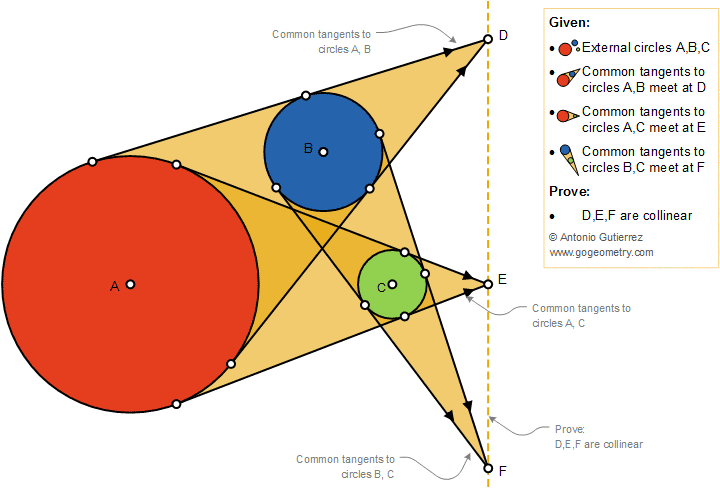# Geometry Problem 1393: Monge's Circle Theorem, Three Circles and Three Pair of Common Tangents, Collinearity

### Proposition

For any three external circles in a plane, the intersection points of each of the three pairs of external tangents are collinear.
Gaspard Monge (1746 - 1818) was a French mathematician, the inventor of descriptive geometry